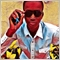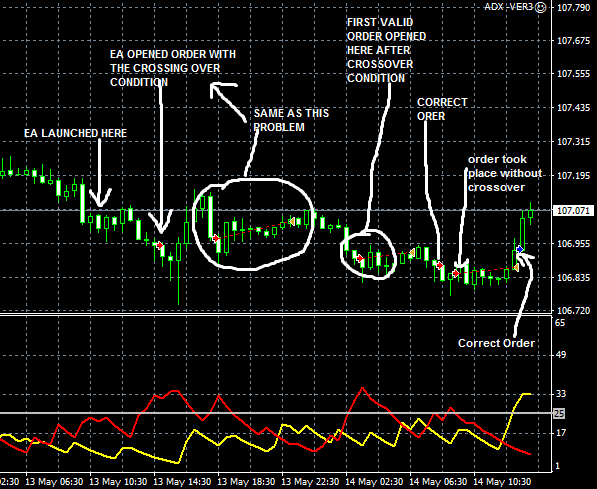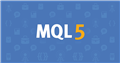# impossible?3699

to skip previous and wait for another condition to happen..

example

if a D- cross above D+ .... condition one

then D- crosses the level line .. condition two

<open a sell order>

the second time D- crosses the level again is ignored..

had to wait for another cross between D- and D+

my code

```input string ADX="================ADX Settings================";
input int Shift=0;

bool a=false,b=false,c=false,d=false,e=false,f=false;

if(dplus> dminus)a=true;
if(a==true && b==true)c=true;
{

}
if(dminus > dplus)d=true;
if(d==true && e==true)f=true;
if(OrdersTotalT(OP_SELL)==0 && f==true )
{
///sellsss///

}

```Documentation on MQL5: Constants, Enumerations and Structures / Trade Constants / Trade Orders in DOM
• www.mql5.com
For equity securities, the Depth of Market window is available, where you can see the current Buy and Sell orders. Desired direction of a trade operation, required amount and requested price are specified for each order. To obtain...3699

Okay got it.. code indicator first.. with 4 buffers..

1st -D cross +D

2nd +D cross -D

3rd -D croses level.

4th +D crosses level..

3 buffers..

If buffer 3 and previous arrow was 1st buffer.. trigger

If buffer 4 and previous arrow was 2nd buffer... trigger3699

now the problem is getting last arrow code..*-

anyone has a solution to get the previous arrow code type?

```double buy=iCustom(NULL, PERIOD_CURRENT, "adx",ADX_Period,ADX_Level,Shift,2,1);
{
}
if(sell!=EMPTY_VALUE && NewBar() && FindLastSell())
{
selling//;..
}

{
double yes=false;
static int offset = 0;
for(int i = ObjectsTotal(OBJ_ARROW)-1; i>= 0; i--)
{
string n = ObjectName(ChartID(), i, -1, OBJ_ARROW);
int code = (int)ObjectGetInteger(ChartID(), n, OBJPROP_ARROWCODE);
if(code == 233)
{
yes=true;
}
}
return yes;
}

double FindLastSell()
{
double yes=false;
for(int i = ObjectsTotal(OBJ_ARROW)-1; i>= 0; i--)
{
string n = ObjectName(ChartID(), i, -1, OBJ_ARROW);
int code = (int)ObjectGetInteger(ChartID(), n, OBJPROP_ARROWCODE);
if(code == 234)
{
yes=true;
}
}
return yes;
}```Documentation on MQL5: Constants, Enumerations and Structures / Indicator Constants / Drawing Styles
• www.mql5.com
When creating a custom indicator, you can specify one of 18 types of graphical plotting (as displayed in the main chart window or a chart subwindow), whose values are specified in the ENUM_DRAW_TYPE enumeration. Depending on the drawing style, you may need one to four value buffers (marked as INDICATOR_DATA). If a style admits dynamic...
Files:3699

Okay have edited the indicator to identify Arrow objects on chart..

since buffer wont print arrow on chart

still not working..

```int FindLastBuyIndex()
{
static int offset = 0;
static int last_i  = -1;
for(int i = ObjectsTotal(OBJ_ARROW)-1; i>= 0; i--)
{
string n = ObjectName(ChartID(), i, -1, OBJ_ARROW);
int code = (int)ObjectGetInteger(ChartID(), n, OBJPROP_ARROWCODE);
if(code == 233)
{
offset = ObjectsTotal(OBJ_ARROW) - i;
//algo2 = true;
last_i = i;
return i;
}
}
return -1;
}

int FindLastSellIndex()
{
for(int i = ObjectsTotal(OBJ_ARROW)-1; i>= 0; i--)
{
string n = ObjectName(ChartID(), i, -1, OBJ_ARROW);
int code = (int)ObjectGetInteger(ChartID(), n, OBJPROP_ARROWCODE);
if(code == 234)
{
return i;
}
}
return -1;
}```
Files:11794

I'm not sure to understand your question ...

```if(dplus> dminus)a=true;
if(a==true && b==true)c=true;
{

}```

is the same as ...

`if((dplus> dminus) && (Cross(0, dplus> ADX_Level) && (OrdersTotalT(OP_BUY)==0)) { // buys }`

... and it means that ...

Did you forgot to reset your booleans variables ?3699

Icham Aidibe:

I'm not sure to understand your question ...

is the same as ...

... and it means that ...

Did you forgot to reset your booleans variables ?

That one works perfectly..

What i want to get is..

If +D Crosses the line for the second time it is ignored.. unless another cross of +D and -D happens..

So i made the indicator to print arrows

For 4 buffers

1st -D cross above +D =color Red

2nd +D cross above -D =color Green

3rd -D croses above level = color yellow

4th +D crosses above level = color aqua

So now if color Aqua apears and previous arrow was Green it should placed a buy

If another aqua apears and previous arrow was not green.. not a buy..

For sell...

If color yellow apears and previous arrow was Red it should place a sell

Am finding it difficult to get previous arrow type.. tried many function..

The only solution i did to bypass it was

If Green apears place a buystop and instantly delete it.. so if Aqua apears it will check if previous closed trade is a buystop.. then place a BUy if not its skipped..

Same for sell

If Red appears place a sell stop and instantly delete it.. so if Yello apears it will check for previous closed trade if its a sellstop.. then place a sell .. if not it s skipped..

This is the only solution i did it worked but not perfect..

Getting the previous arrow code will work perfectly.. i tried different codes.. none worked

https://www.mql5.com/en/forum/341195